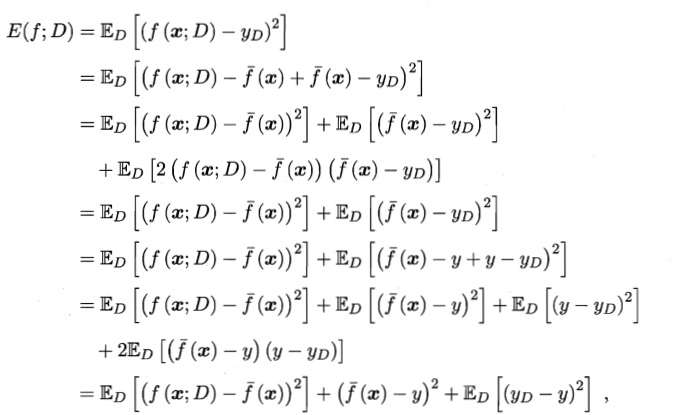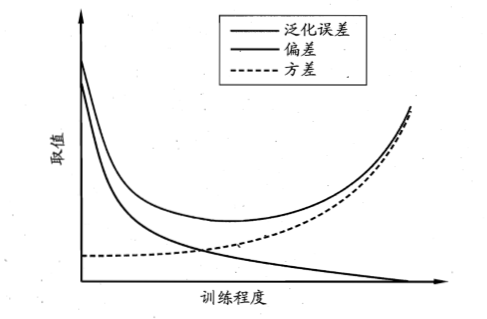• 算法期望泛化误差算法期望泛化误差 （本文为个人学习总结笔记） 算法期望泛化误差 原公式： E(f;D)=ED[(f(x;D)−yD)2]=ED[(f(x;D)−fˉ(x)+fˉ(x)−yD)2]=ED[(f(x;D)−fˉ(x))2]+ED[(fˉ(x)−yD)2]+ED[+2(f(x;D)−f...
算法期望泛化误差算法期望泛化误差
（本文为个人学习总结笔记）
算法期望泛化误差
原公式：
\begin{aligned} E(f ; D)=& \mathbb{E}_{D}\left[\left(f(\boldsymbol{x} ; D)-y_{D}\right)^{2}\right] \\ =& \mathbb{E}_{D}\left[\left(f(\boldsymbol{x} ; D)-\bar{f}(\boldsymbol{x})+\bar{f}(\boldsymbol{x})-y_{D}\right)^{2}\right] \\ =& \mathbb{E}_{D}\left[(f(\boldsymbol{x} ; D)-\bar{f}(\boldsymbol{x}))^{2}\right]+\mathbb{E}_{D}\left[\left(\bar{f}(\boldsymbol{x})-y_{D}\right)^{2}\right] \\ &+\mathbb{E}_{D}\left[+2(f(\boldsymbol{x} ; D)-\bar{f}(\boldsymbol{x}))\left(\bar{f}(\boldsymbol{x})-y_{D}\right)\right] \\ =& \mathbb{E}_{D}\left[(f(\boldsymbol{x} ; D)-\bar{f}(\boldsymbol{x}))^{2}\right]+\mathbb{E}_{D}\left[\left(\bar{f}(\boldsymbol{x})-y_{D}\right)^{2}\right] \\ =& \mathbb{E}_{D}\left[(f(\boldsymbol{x} ; D)-\bar{f}(\boldsymbol{x}))^{2}\right]+\mathbb{E}_{D}\left[\left(\bar{f}(\boldsymbol{x})-y+y-y_{D}\right)^{2}\right] \\ =& \mathbb{E}_{D}\left[(f(\boldsymbol{x} ; D)-\bar{f}(\boldsymbol{x}))^{2}\right]+\mathbb{E}_{D}\left[\left(\bar{f}(\boldsymbol{x})-y+\mathbb{E}_{D}\left[\left(y-y_{D}\right)^{2}\right]\right.\right.\\ &+2 \mathbb{E}_{D}\left[(\bar{f}(\boldsymbol{x})-y)\left(y-y_{D}\right)\right] \\ =& \mathbb{E}_{D}\left[(f(\boldsymbol{x} ; D)-\bar{f}(\boldsymbol{x}))^{2}\right]+(\bar{f}(\boldsymbol{x})-y)^{2}+\mathbb{E}_{D}\left[\left(y_{D}-y\right)^{2}\right] \end{aligned}
1、第一步：减一个$\bar{f}(\boldsymbol{x})$再加一个$\bar{f}(\boldsymbol{x})$，属于简单的恒等变形。
2、第二步：首先将中括号中的式子展开
$\mathbb{E}_{D}\left[(f(\boldsymbol{x} ; D)-\bar{f}(\boldsymbol{x}))^{2}+\left(\bar{f}(\boldsymbol{x})-y_{D}\right)^{2}+2(f(\boldsymbol{x} ; D)-\bar{f}(\boldsymbol{x}))\left(\bar{f}(\boldsymbol{x})-y_{D}\right)\right]$
然后根据期望的运算性质，得：
$\mathbb{E}_{D}\left[(f(\boldsymbol{x} ; D)-\bar{f}(\boldsymbol{x}))^{2}\right]+\mathbb{E}_{D}\left[\left(\bar{f}(\boldsymbol{x})-y_{D}\right)^{2}\right]+\mathbb{E}_{D}\left[2(f(\boldsymbol{x} ; D)-\bar{f}(\boldsymbol{x}))\left(\bar{f}(\boldsymbol{x})-y_{D}\right)\right]$
3、第三步：再次利用期望的运算性质将第3步得到的式子的最后一项展开
\begin{aligned} &\mathbb{E}_{D}\left[2(f(\boldsymbol{x} ; D)-\bar{f}(\boldsymbol{x}))\left(\bar{f}(\boldsymbol{x})-y_{D}\right)\right]=\mathbb{E}_{D}[2(f(\boldsymbol{x} ; D)-\bar{f}(\boldsymbol{x})) \cdot \bar{f}(\boldsymbol{x})]-\mathbb{E}_{D}\left[2(f(\boldsymbol{x} ; D)-\bar{f}(\boldsymbol{x})) \cdot y_{D}\right]\\ &=0+0 \end{aligned}
4、第四步：同第1步一样，减一个y再加一个y，属于简单的恒等变形；
5、第五步：同第2步一样，将最后一项利用期望的运算性质进行展开；
6、第六步：因为$\bar{f}(\boldsymbol{x})$和y均为常量，所以根据期望的运算性质可知，第6步中的第2项可化为
$\mathbb{E}_{D}\left[(\bar{f}(\boldsymbol{x})-y)^{2}\right]=(\bar{f}(\boldsymbol{x})-y)^{2}$
同理，第6步中的最后一项可化为：
$2 \mathbb{E}_{D}\left[(\bar{f}(\boldsymbol{x})-y)\left(y-y_{D}\right)\right]=2(\bar{f}(\boldsymbol{x})-y) \mathbb{E}_{D}\left[\left(y-y_{D}\right)\right]$
由于此时假设噪声的期望为零，故：
$2 \mathbb{E}_{D}\left[(\bar{f}(\boldsymbol{x})-y)\left(y-y_{D}\right)\right]=2(\bar{f}(\boldsymbol{x})-y) \cdot 0=0$


展开全文• 一、期望泛化误差的偏差-方差分解 偏差-方差分解试图对机器学习算法的期望泛化误差率进行拆解。 记为测试样本，为训练集D上学习得到的模型在上的预测输出，为在数据集中的标记，为的真实标记。 对算法的期望泛化...
一、期望泛化误差的偏差-方差分解

偏差-方差分解试图对机器学习算法的期望泛化误差率进行拆解。

记为测试样本，为训练集D上学习得到的模型在上的预测输出，为在数据集中的标记，为的真实标记。

对算法的期望泛化误差进行分解：得到：

即泛化误差可分解为偏差、方差与噪声之和。其中偏差度量了学习算法的期望预测与真实结果的偏离程度，即刻画了学习算法本身的拟合能力；方差度量了同样大小的训练集的变动所导致的学习性能的变化，即刻画了数据扰动所造成的影响；噪声表达了在当前任务上任何学习算法所能达到的期望泛化误差的下界，即刻画了学习问题本身的难度。偏差-方差分解说明，泛化性能是由学习算法的能力、数据的充分性以及学习任务本身的难度共同决定的。

二、偏差-方差窘境（bias-variance dilemma）

给定学习任务，如果我们能控制学习算法的训练程度，则在训练程度不足的时候，学习器的拟合能力不够，训练数据的扰动不足以使学习器产生显著变化，此时偏差主导了泛化错误率；随着训练程度的加深，学习器的拟合能力逐渐加强，训练数据的扰动渐渐能被学习器学到，方差逐渐住到了泛化错误率；在训练程度充足后，学习器的拟合能力已经非常强，训练数据的轻微扰动都能导致学习器的显著变化。若训练数据自身的，非全局的特性被学习器学到了，则将发生过拟合。

泛化误差与偏差、方差的关系示意图如下所示：展开全文机器学习
• 偏差度量了学习算法的期望预测与真实结果的偏离程度，即刻画了学习...噪声则表达了在当前任务上任何学习算法所能达到的期望泛化误差的下界，即刻画了学习问题本身的难度 泛化误差可分解为偏差、方差与噪声之和. ...
偏差度量了学习算法的期望预测与真实结果的偏离程度，即刻画了学习算法本身的拟合能力
方差度量了同样大小的训练集的变动所导致的学习性能的变化，即刻画了数据扰动所造成的影响
噪声则表达了在当前任务上任何学习算法所能达到的期望泛化误差的下界，即刻画了学习问题本身的难度
泛化误差可分解为偏差、方差与噪声之和.


展开全文• 经验误差，泛化误差 前言我们在上篇博文 《机器学习模型的容量，过拟合与欠拟合》 中曾经提到过模型的泛化问题，指的就是描述一个模型在未见过的数据中的表现能力。这里再提出了，用于比较经验误差。 联系方式： ...

经验误差，泛化误差

前言
我们在上篇博文 《机器学习模型的容量，过拟合与欠拟合》 中曾经提到过模型的泛化问题，指的就是描述一个模型在未见过的数据中的表现能力。这里再提出了，用于比较经验误差。联系方式：e-mail: FesianXu@163.comQQ: 973926198github: https://github.com/FesianXu
假设我们现在有数据集D={(x1,y1),(x2,y2),⋯ ,(xi,yi)},i=ND=\{(x_1,y_1), (x_2,y_2),\cdots,(x_i,y_i)\}, i=N,其中NN是数据集的大小，xix_i为数据的属性1，yiy_i为标签。假设有yi∈Yy_i \in \mathcal{Y}，xi∈X,i=1,2,⋯ ,Nx_i \in \mathcal{X}, \rm i =1,2,\cdots,N，假设X\mathcal{X}中的所有样本都满足一个隐含的，未知的分布D\mathcal{D}，也就是说DD中的所有样本都是从D\mathcal{D}中独立同分布(i.i.d) 地采样的。
然后假设hh是算法L\mathcal{L}学习到的从X\mathcal{X}到Y\mathcal{Y}的映射，y=h(x)y=h(x)，并且有h∈Hh \in\mathcal{H}，其中H\mathcal{H}为算法L\mathcal{L}的假设空间。我们可以定义映射 hh 的 泛化误差(generalization error):
E(h;D)=Px∼D(h(x)≠y)(1.1)
E(h; \mathcal{D}) = \rm P_{x \sim \mathcal{D}} \rm(h(x) \neq y)
\tag{1.1}

因为我们无法观察到整个分布D\mathcal{D}，只能观察到独立同分布采样后的DD，因此我们需要定义 经验误差(empirical error):E^(h;D)=1N∑i=1N1(h(xi)≠yi),xi∈D(1.2)
\hat E(h;\mathcal{D}) = \rm \frac{1}{N} \sum_{i=1}^N 1(h(x_i) \neq y_i),x_i \in D
\tag{1.2}

其中的1(⋅)1(\cdot)表示当条件符合时输出1，否则输出0。由于DD是D\mathcal{D}的独立同分布采样，因此hh的经验误差的期望等于泛化误差。
引用：
《机器学习模型的容量，过拟合与欠拟合》 CSDN
《机器学习（四）经验风险与结构风险》 CSDN
《机器学习》 周志华著
数据的属性指的是数据的最原始的特征，比如图片的原始像素点，而数据的特征大多指的是属性经过特定的操作的数据，如图片的像素点经过CNN卷积之后得到的特征。广义来说，数据的属性和特征没有区别。 ↩︎


展开全文机器学习 深度学习 人工智能
• 误差（error）：一般地，我们把学习器的实际预测输出与样本的真是输出之间的差异称为“误差” 经验误差（empirical ...泛化误差（generalization error）：模型在新样本集（测试集）上的误差称为“泛化误差”。 ...
• 泛化能力 一、 泛化误差 学习方法的泛化能力（generalization ability）：方法学习到的模型对未知数据...泛化误差定义：如果学习到的模型是f^\hat ff^​，那么用这个模型对未知数据预测的误差即为泛化误差（generali...机器学习 统计学
• 个人对泛化误差的看法   泛化误差与交叉验证 误差这个词我们经常会遇到，在机器学习中，我们最终想要的结果实际上就是减小学习后的估计值和真实值的误差。比如在回归中，我们的 loss function 就表示一个误差。...交叉验证
• 今天我就来说说训练误差、测试误差、泛化误差到底是什么，区别所在。 对于分类学习算法，我们一般将样本集分为训练集和测试集，其中训练集用于算法模型的学习或训练，而测试集通常用于评估训练好的模型对于数据的...训练误差 测试误差
• 我们需要区分训练误差（training error）和泛化误差（generalization error）。通俗来讲，前者指模型在训练数据集上表现出的误差，后者指模型在任意一个测试数据样本上表现出的误差的期望，并常常通过测试数据集上的...机器学习 深度学习 K折交叉验证
• 泛化误差也可以理解为期望风险，而右式第一个也叫做经验风险。 这都是与我们的的模型相关的，我们希望我们的模型对未知数据也能有好的预测能力，也就是泛化能力较强，这样才能说明我们的模型有着一定的可用性。 ...
• 泛化误差就是模型的期望风险 Rexp(f^)=Ep[L(Y,f^(X))]=∫X∗YL(y,f^(x))P(x,y)dxdyR_{exp}(\hat{f})=E_p[L(Y,\hat{f}(X))]=\int_{\mathcal{X}*\mathcal{Y}}L(y,\hat{f}(x))P(x,y)dxdyRexp​(f^​)=Ep​[L(Y,f^​(X))...机器学习
• 后来业界就提出了泛化误差的概念（generalization error）,在机器学习中泛化误差是用来衡量一个学习机器推广未知数据的能力，即根据从样本数据中学习到的规则能够应用到新数据的能力。常用的计算方法是：用在训练集...
• 【统计学习笔记】泛化误差上界1. 泛化误差2. 泛化误差上界 1. 泛化误差 学习方法的泛化能力是指由该方法学习到的模型对未知数据的预测能力，是学习方法本质上重要的性质。测试误差是依赖于测试数据集的，泛化误差是...机器学习
• 泛化误差 = 偏差的平方 + 方差 + 噪声 偏差度量了真实数据与预测数据的偏离程度，刻画了学习算法本身的拟合能力 方差度量了同样大小的训练集的变动所导致的学习性能的变化，刻画了数据扰动所带来的影响； ...
• 泛化误差（generalization error）：模型在任意一个测试数据样本上表现出的误差的期望，并常常通过测试数据集上的误差来近似 总结 一味地降低训练误差并不意味着泛化误差一定会降低。机器学习模型应关注降低泛化误差...深度学习 机器学习 神经网络 人工智能
• 经验误差与泛化误差、偏差与方差、欠拟合与过拟合、交叉验证 主要内容 经验误差与泛化误差 偏差与方差 欠拟合与过拟合 交叉验证 一、经验误差（训练误差）与泛化误差   经验误差（训练误差）：模型在训练集上的...机器学习
• 括号内是具体内容loge : 1.loge =lne =1 2.loge=lge=log(e) = 0.43429448190324 (摘自百度 : )笔者在一个公式上因为这个卡了15分钟）好了，先给出书上定义：不等式（1.25）左端R（f）是泛化误差，右端即为泛化误差...
• 泛化误差：模型是f^\hat{f}f^​，该模型对于未知数据预测的误差即为泛化误差： Rexp⁡(f^)=EP[L(Y,f^(X))]=∫X×YL(y,f^(x))P(x,y)dx dy \begin{aligned} R_{\exp }(\hat{f}) &=E_{P}[L(Y, \hat{f}(X))] \\...机器学习
• 一般而言，我们将考虑训练集上的训练误差和测试集上的泛化误差，事实上，训练误差的持续降低并不是那么令人愉快，因为这可能是“过拟合”在背后操纵着一切。总的来说，只有泛化误差的降低才能真的让人感觉美滋滋。 ...
• 机器学习算法的最终目标是最小化...我们希望所学习到的该模型对未知数据预测的误差尽可能小，这里的误差我们就将其定义为机器学习算法的泛化误差（generalization error）：Rexp⁡(f^)=EP[L(Y,f^(X))]=∫X×YL(y,f^(x)训练误差 测试误差
• 本文主要参考书籍为《统计学习方法》（李辉），第一章 统计学习方法概论。1.6 泛化能力1.6.1 泛化误差评价模型对未知数据的预测能力。现实中采用最多的办法是依赖...泛化误差就是模型的期望风险。1.6.2 泛化误差上届...机器学习
• 泛化 　是机器学习本身的核心。简单说，泛化就是在训练数据集上训练好的模型，在测试数据集上表现如何。 　正则化”是我们用来防止过拟合的技术。由于我们没有任何关于测试扰动的先验信息，所以通常我们所能做...
• 这就是机器学习中的“模型选择”问题，理想的解决方案是对候选模型的泛化误差进行评估，然后选择泛化误差最小的那个模型。 泛化误差意义 以前在机器学习中一直使用经验风险（训练误差）来逼近真实风险，但事实上...噪声
• 泛化误差上界： 对二分类问题，当假设空间是有限个函数的集合F={f1,f2,f3,...,fn}时，对任意一个函数，至少以概率，以下不等式成立： 其中， 不等式右端第一项为训练误差，训练误差越小，泛化误差就越小 第二...机器学习...